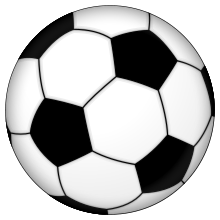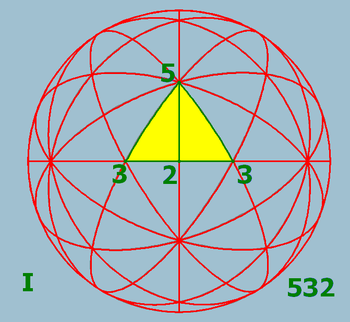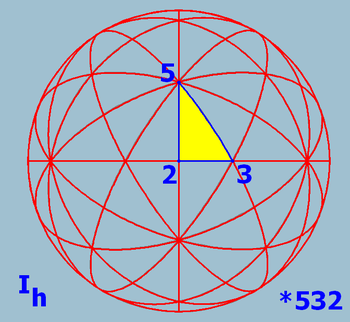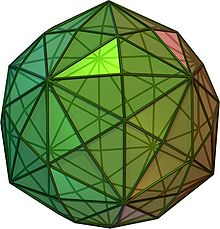# Icosahedral symmetry

Icosahedral symmetryA Soccer ball, a common example of a spherical truncated icosahedron, has full icosahedral symmetry.

A regular icosahedron has 60 rotational (or orientation-preserving) symmetries, and a symmetry order of 120 including transformations that combine a reflection and a rotation. A regular dodecahedron has the same set of symmetries, since it is the dual of the icosahedron.

The set of orientation-preserving symmetries forms a group referred to as A5 (the alternating group on 5 letters), and the full symmetry group (including reflections) is the product A5 × C2. The latter group is also known as the Coxeter group H3.

## As point groupThe icosahedral rotation group I with fundamental domain

Apart from the two infinite series of prismatic and antiprismatic symmetry, rotational icosahedral symmetry or chiral icosahedral symmetry of chiral objects and full icosahedral symmetry or achiral icosahedral symmetry are the discrete point symmetries (or equivalently, symmetries on the sphere) with the largest symmetry groups.

Icosahedral symmetry is not compatible with translational symmetry, so there are no associated crystallographic point groups or space groups.

Schönflies
crystallographic
notation
Coxeter
notation
Orbifold
notation
Order
I [3,5]+ 532 60
Ih [3,5] *532 120

Presentations corresponding to the above are:$I: \langle s,t \mid s^2, t^3, (st)^5 \rangle\$$I_h: \langle s,t\mid s^3(st)^{-2}, t^5(st)^{-2}\rangle.\$

These correspond to the icosahedral groups (rotational and full) being the (2,3,5) triangle groups.

The first presentation was given by William Rowan Hamilton in 1856, in his paper on Icosian Calculus.

Note that other presentations are possible, for instance as an alternating group (for I).

## Group structure

The icosahedral rotation group I is of order 60. The group I is isomorphic to A5, the alternating group of even permutations of five objects. This isomorphism can be realized by I acting on various compounds, notably the compound of five cubes (which inscribe in the dodecahedron), the compound of five octahedra, or either of the two compounds of five tetrahedra (which are enantiomorphs, and inscribe in the dodecahedron).

The group contains 5 versions of Th with 20 versions of D3 (10 axes, 2 per axis), and 6 versions of D5.

The full icosahedral group Ih has order 120. It has I as normal subgroup of index 2. The group Ih is isomorphic to I × C2, or A5 × C2, with the inversion in the center corresponding to element (identity,-1), where C2 is written multiplicatively.

Ih acts on the compound of five cubes and the compound of five octahedra, but -1 acts as the identity (as cubes and octahedra are centrally symmetric). It acts on the compound of ten tetrahedra: I acts on the two chiral halves (compounds of five tetrahedra), and -1 interchanges the two halves. Notably, it does not act as S5, and these groups are not isomorphic; see below for details.

The group contains 10 versions of D3d and 6 versions of D5d (symmetries like antiprisms).

I is also isomorphic to PSL2(5), but Ih is not isomorphic to SL2(5).

### Commonly confused groups

The following groups all have order 120, but are not isomorphic:

They correspond to the following short exact sequences (which do not split) and product$1\to A_5 \to S_5 \to C_2 \to 1$$I_h = A_5 \times C_2$$1\to C_2 \to 2I\to A_5 \to 1$

In words,

Note that A5 has an exceptional irreducible 3-dimensional representation (as the icosahedral rotation group), but S5 does not have an irreducible 3-dimensional representation, corresponding to the full icosahedral group not being the symmetric group.

These can also be related to linear groups over the finite field with five elements, which exhibit the subgroups and covering groups directly; none of these are the full icosahedral group:

•$A_5 \cong \operatorname{PSL}(2,5),$ the projective special linear group, see here for a proof;
•$S_5 \cong \operatorname{PGL}(2,5),$ the projective general linear group;
•$2I \cong \operatorname{SL}(2,5),$ the special linear group.

### Conjugacy classes

The conjugacy classes of I are:

• identity
• 12 × rotation by 72°, order 5
• 12 × rotation by 144°, order 5
• 20 × rotation by 120°, order 3
• 15 × rotation by 180°, order 2

Those of Ih include also each with inversion:

• inversion
• 12 × rotoreflection by 108°, order 10
• 12 × rotoreflection by 36°, order 10
• 20 × rotoreflection by 60°, order 6
• 15 × reflection, order 2

### Subgroups

• Ih,I , Th and T
• D2h
• D5d, D3d
• D5, D3 and D2
• C2h
• C5v, C3v and C2v
• C5, C3 and C2
• S10, S6 and S2=Ci
• E and Cs

All of these classes of subgroups are conjugate (i.e., all vertex stabilizers are conjugate), and admit geometric interpretations.

Note that the stabilizer of a vertex/edge/face/polyhedron and its opposite are equal, since − 1 is central.

#### Vertex stabilizers

Stabilizers of an opposite pair of vertices can be interpreted as stabilizers of the axis they generate.

• vertex stabilizers in I give cyclic groups C3
• vertex stabilizers in Ih give dihedral groups D3
• stabilizers of an opposite pair of vertices in I give dihedral groups D3
• stabilizers of an opposite pair of vertices in Ih give$D_3 \times \pm 1$

#### Edge stabilizers

Stabilizers of an opposite pair of edges can be interpreted as stabilizers of the rectangle they generate.

• edges stabilizers in I give cyclic groups C2
• edges stabilizers in Ih give Klein four-groups$C_2 \times C_2$
• stabilizers of a pair of edges in I give Klein four-groups$C_2 \times C_2$; there are 5 of these, given by rotation by 180° in 3 perpendicular axes.
• stabilizers of a pair of edges in Ih give$C_2 \times C_2 \times C_2$; these are 5 of these, given by reflections in 3 perpendicular axes.

#### Face stabilizers

Stabilizers of an opposite pair of faces can be interpreted as stabilizers of the anti-prism they generate.

• face stabilizers in I give cyclic groups C5
• face stabilizers in Ih give dihedral groups D5
• stabilizers of an opposite pair of faces in I give dihedral groups D5
• stabilizers of an opposite pair of faces in Ih give$D_5 \times \pm 1$

#### Polyhedron stabilizers

For each of these, there are 5 conjugate copies, and the conjugation action gives a map, indeed an isomorphism,$I \stackrel{\sim}\to A_5 < S_5$.

• stabilizers of the inscribed tetrahedra in I are a copy of T
• stabilizers of the inscribed tetrahedra in Ih are a copy of Th
• stabilizers of the inscribed cubes (or opposite pair of tetrahedra, or octahedrons) in I are a copy of O
• stabilizers of the inscribed cubes (or opposite pair of tetrahedra, or octahedrons) in Ih are a copy of Oh

## Fundamental domain

Fundamental domains for the icosahedral rotation group and the full icosahedral group are given by:The icosahedral rotation group I with fundamental domainThe full icosahedral group Ih with fundamental domainFundamental domain in the disdyakis triacontahedron

In the disdyakis triacontahedron one full face is a fundamental domain; other solids with the same symmetry can be obtained by adjusting the orientation of the faces, e.g. flattening selected subsets of faces to combine each subset into one face, or replacing each face by multiple faces, or a curved surface.

## Solids with icosahedral symmetry

### Full icosahedral symmetry

Platonic solids - regular polyhedra (all faces of the same type)

Archimedean solids - polyhedra with more than one polygon face type.

Catalan solids - duals of the Archimedean solids.

## Other objects with icosahedral symmetry

• Barth surfaces
• Virus structure, and Capsid

## Liquid Crystals with icosahedral symmetry

For the intermediate material phase called Liquid Crystals the existence of icosahedral symmetry was proposed by H. Kleinert and K. Maki and its structure was first analyzed in detail in that paper. See the review article here. In aluminum, the icohahedral structure was discovered experimentally three years after this by Dan Shechtman, which earned him the Nobel Prize in 2011.

## Related geometries

Icosahedral symmetry is equivalently the projective special linear group PSL(2,5), and is the symmetry group of the modular curve X(5), and more generally PSL(2,p) is the symmetry group of the modular curve X(p). The modular curve X(5) is geometrically a dodecahedron with a cusp at the center of each polygonal face, which demonstrates the symmetry group.

This geometry, and associated symmetry group, was studied by Felix Klein as the monodromy groups of a Belyi surface – a Riemann surface with a holomorphic map to the Riemann sphere, ramified only at 0, 1, and infinity (a Belyi function) – the cusps are the points lying over infinity, while the vertices and the centers of each edge lie over 0 and 1; the degree of the covering (number of sheets) equals 5.

This arose from his efforts to give a geometric setting for why icosahedral symmetry arose in the solution of the quintic equation, with the theory given in the famous (Klein 1888); a modern exposition is given in (Tóth 2002, Section 1.6, Additional Topic: Klein's Theory of the Icosahedron, p. 66).

Klein's investigations continued with his discovery of order 7 and order 11 symmetries in (Klein 1878/79b) and (Klein 1879) (and associated coverings of degree 7 and 11) and dessins d'enfants, the first yielding the Klein quartic, whose associated geometry has a tiling by 24 heptagons (with a cusp at the center of each).

Similar geometries occur for PSL(2,n) and more general groups for other modular curves.

More exotically, there are special connections between the groups PSL(2,5) (order 60), PSL(2,7) (order 168) and PSL(2,11) (order 660), which also admit geometric interpretations – PSL(2,5) is the symmetries of the icosahedron (genus 0), PSL(2,7) of the Klein quartic (genus 3), and PSL(2,11) the buckyball surface (genus 70). These groups form a "trinity" in the sense of Vladimir Arnold, which gives a framework for the various relationships; see trinities for details.

There is a close relationship to other Platonic Solids.

## See also

Wikimedia Foundation. 2010.

### Look at other dictionaries:

• icosahedral symmetry — an arrangement of viral subunits in which the structure of the viral capsid is characterized by symmetry having the rotation axes of a regular polygon with 20 triangular surfaces (icosahedron); each face contains several subunits, the total… …   Medical dictionary

• Solids with icosahedral symmetry — olids with full icosahedral symmetry (For details see below.) Platonic solids regular polyhedra (all faces of the same type) {5,3} {3,5}Archimedean solids polyhedra with more than one polygon face type. 3.10.10 4.6.10 5.6.6 3.4.5.4 3.5.3.5Catalan …   Wikipedia

• icosahedral — Having 20 equilateral triangular surfaces and 12 vertices, as do most viruses with cubic symmetry. [G. eikosi, twenty, + edros, having sides or bases] * * * ico·sa·he·dral (i″ko sə heґdrəl) [Gr. eikosi twenty + hedra seat]… …   Medical dictionary

• Tetrahedral symmetry — A regular tetrahedron has 12 rotational (or orientation preserving) symmetries, and a total of 24 symmetries including transformations that combine a reflection and a rotation.The group of symmetries that includes reflections is isomorphic to S 4 …   Wikipedia

• List of spherical symmetry groups — List of symmetry groups on the sphere = Spherical symmetry groups are also called point groups in three dimensions. This article is about the finite ones.There are four fundamental symmetry classes which have triangular fundamental domains:… …   Wikipedia

• Octahedral symmetry — The cube is the most common shape with octahedral symmetry A regular octahedron has 24 rotational (or orientation preserving) symmetries, and a symmetry order of 48 including transformations that combine a reflection and a rotation. A cube has… …   Wikipedia

• Binary icosahedral group — In mathematics, the binary icosahedral group is an extension of the icosahedral group I of order 60 by a cyclic group of order 2. It can be defined as the preimage of the icosahedral group under the 2:1 covering homomorphism:mathrm{Sp}(1) o… …   Wikipedia

• Molecular symmetry — in chemistry describes the symmetry present in molecules and the classification of molecules according to their symmetry. Molecular symmetry is a fundamental concept in chemistry, as it can predict or explain many of a molecule s chemical… …   Wikipedia

• binal symmetry — The symmetry of some virus capsids (e.g., those of complex phages) that is a combination of icosahedral and helical symmetry …   Dictionary of microbiology

• Point groups in three dimensions — In geometry, a point group in three dimensions is an isometry group in three dimensions that leaves the origin fixed, or correspondingly, an isometry group of a sphere. It is a subgroup of the orthogonal group O(3), the group of all isometries… …   Wikipedia

### Share the article and excerpts

##### Direct link
Do a right-click on the link above
and select “Copy Link”